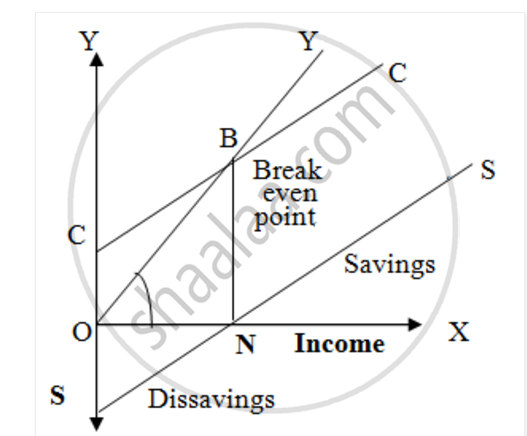# Explain in Detail ‘Saving Function’ with Schedule and Diagram. - Economics

Explain in detail ‘saving function’ with schedule and diagram.

#### Solution

Meaning:- The saving function is also called as propensity to save. The saving function is the counterpart (matching part) of the consumption function. The amount of saving at any level of income is equal to the difference between the income and consumption expenditure.

It can be expressed as follows:-

S = Y – C

The relationship between income and saving is shown in the diagram.In the above diagram, ‘SS’ curve is the saving curve, which is the counterpoint of consumption curve ‘CC’.

At ‘ON’ level of income, consumption equals income at point ‘B’ and the savings is zero. When income is lower than ‘ON’, consumption is greater than income and there isdis-saving because consumption is done through borrowing. So saving curve ‘SS’ is below ‘OX’ –axis. When income is greater than ‘ON’, the consumption is lower than income and there is savings. So, saving curve ‘SS’ is above OX-axis.the propensity to save depends on the level of income. The relationship between savings and income is direct and positive.

Normally, higher the income, higher would be the saving. Therefore, saving is the function of income. It can be expressed as follows:-

S=f (Y)

Saving Schedule:-

The relationship between income and saving can be shown in the following schedule.

Saving Schedule (Rs. Crore)

 Income (Y) Consumption (C) Savings (S) 10000 13000 -3000 15000 15000 Zero 20000 17000 3000 25000 19000 6000 30000 21000 9000

From the above schedule, it is clear that there is direct and positive relationship between income and saving. As the income increases, savings also increase depending upon the consumption expenditure.

Concept: Concept of National Income
Is there an error in this question or solution?
2014-2015 (March)

Share